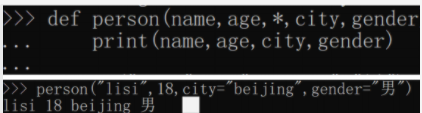# python 命名关键字参数 参数组合 参数小结

## 9.5.5 命名关键字参数

def person(name,age,*,city,job)

def person(name,age,city)

，*后面的参数被视为命名关键字参数。## 9.5.6 参数的组合

def  func(a,b,c=0,*args,**kw):
print 'a=',a,'b=',b,'c=',c,'args=',args,'kw=',kw

print  func(1,2)
# = > a= 1 b= 2 c= 0 args= () kw= {}

print func(1,2,c=3)
# = > a= 1 b= 2 c= 3 args= () kw= {}

print func(1,2,3,'a','b')
# => a= 1 b= 2 c= 3 args= ('a', 'b') kw= {}

print  func(1,2,3,'a','b',x=99)
# =>a= 1 b= 2 c= 3 args= ('a', 'b') kw= {'x': 99}


## 小结

06-051万+08-222099
12-094684
01-24615
04-131万+
08-0365
07-10530
10-08714
01-191082
08-31135
03-26205
03-1577
06-11469
04-03366
06-1546
11-1687
12-26223
©️2020 CSDN 皮肤主题: 护眼 设计师:闪电赇